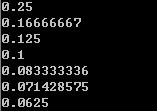## Saturday, 28 February 2015

### Review 4.2: Document filtering - Calculate probabilities and Make a reasonable guess

Document filtering: Calculate probabilities and Make a reasonable guess

Firstly, we will talk about conditional probabilites to classify a item. Pls note that we assume that one feature always appears once in a item. So the count of features in a category will not be larger than the number of items in the category. Therefore, We can calculate the probability that a feature accures in a category by using the following forumla:

Probability = featureCountInCategory / itemCountInThisCategory

Next, let us talk about the reason to make a reasonable guess. Take a look the following example
train("Nobody owns the water.","good")
train("the quick rabbit jumps fences","good")
train("make quick money at the online casino","bad")
train("the quick brown fox jumps","good")

We find the feature "money" only appear once in all training samples. And the item, which contains "money", is trainned to be "bad". But in real world, money is not directly linked to bad thingy. So , if we have little information about a feature, we need to make some reasonable guess:
WeightedProbability = (weight * assumeProbility + totalFeaturesInAllCategory * ProbabilityOfTheFeature) / (weight +totalFeaturesInAllCategory)
[As an outcome of experience, weight can be 1.0 and assumeProbability can be 0.5.]

Here is the code of Calculating probabilites and Making a reasonable guess, and the way how the reasonable guess affects the result will be explained later:

```def train(item:String, cate:String) {
getFeatures(item).foreach(incf(_,cate))
incc(cate)
}

def getWeightedProb(feature:String, cate:String , weight:Float = 1.0f, assumeProb:Float = 0.5f) = {
val basicProb = this.getFeatureProb(feature,cate)
val total = this.fc(feature).values.sum

(weight * assumeProb + basicProb * total) / (total + weight)
}

def getFeatureProb(feature:String , cate:String) = countItemInCate(cate) match {
case 0 ⇒ 0
case _ ⇒ countFeatureInCate(feature,cate).toFloat / countItemInCate(cate).toFloat
}

```

A set of training samples:The result:The result shows that how a reasonable guess affects the result: As the information about a feature grows, the result are pulled a lot more far away from the assumed probability.